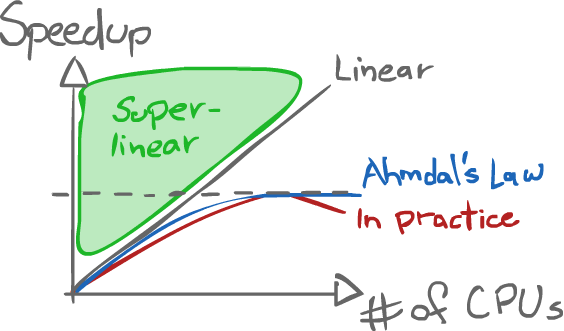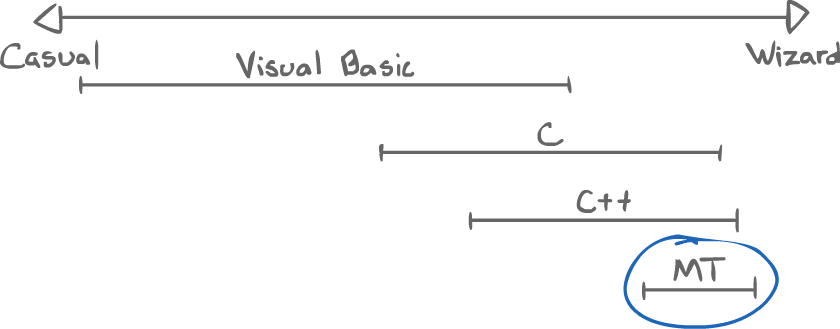Wednesday, September 4, 2013What is concurrency?

1What is concurrency?¶

1.1Parallelism¶

Things happen at the same time

• Multiple processes
• User-controlled, directed
• Interaction is fully determined

1.1.1Metaphor of a Sports team¶

• The user is the coach
• The team's players are the processes
• The coach gives a precise plan for the players to follow
• Cooperative

1.2Concurrent Programming¶

Things might happen at the same time

• User initiates processes but has no precise control
• Barriers and constraints are added to achieve indirect control

1.2.1Sports team metaphor¶

• The programmer is the referee
• The referee simply says the rules of the game
• Competitive

2.1Processes¶

A process is large and heavyweight, a major task.
It has:

• its own I/O
• ...

Context switching is pretty slow, because of all the exclusivity.

Inter-process communication is possible thanks to:

• Pipes
• File System
• Shared memory
• etc.

• Shared file handles

As such, context switching is fast.

Communication amongst threads is easy and fast.

2.2.1Issues¶

• Asynchronous: no direct control on the speed
• Synchronization: the way to set up rules/barriers is not always obvious
• Resource-consistency
• Fairness

3.1Lightweight processes (~ LWP)¶

"An independent flow of control within a process composed of a context (including registers and program counter) and a sequence of instructions to execute."

3.2Role of the OS¶

• Apply a scheduling policy (which may be unique to threads)
• Honor signal masks (thus allowing certain signals to be ignored)

3.3.1Things they own¶

• Registers
• Program counter
• Stack pointer (each thread has its own stack)

3.3.2Things they share¶

• Code space (they come from the same program)
• Global/static data
• Heap, dynamic data (allocated memory)
• File handles

3.4Scheduling issues¶

The OS may or may not schedule threads at the same time or concurrently.

It is our role to make programs correct, no matter how the OS handles threads.

1-to-1
Create a thread; create a process
"True concurrency" can be achieved this way
n-to-1
All threads are in one process
n-to-n
The OS decides
Some threads are mapped to some processes

3.6.1Speedup: Amdahl's Law¶

$$t = s + \frac{p}{n}$$
Where:

• $t$: Total time
• $s$: Time of the sequential part
• $p$: Time of the parallel part
• $n$: Number of processors

The measure of speedup is calculated as the following ratio:
$$\text{Speedup} = \frac{\text{old time}}{\text{new time}}$$

Amdahl's Law can also be normalized:
$$\begin{equation} \begin{split} \text{Let } t &= 1 \\ \text{ie } s+p &= 1 \\ s &= 1-p \\ p &= 1-s \\ \text{Speedup} &= \frac{1}{(1-p) + \frac{p}{n}} \end{split} \end{equation}$$

3.6.1.1Example¶

Say $75\%$ of a program is parallelizable.
$$\text{Speedup} = \frac{1}{0.25 + \frac{0.75}{n}}$$

$$\begin{equation} \begin{split} \text{Let } n = 1 &\Rightarrow \text{Speedup } = 1 \\ n = 3 &\Rightarrow \text{Speedup } = 2 \\ n = 75 &\Rightarrow \text{Speedup } = \frac{1}{0.26} \\ n \rightarrow \infty &\Rightarrow \text{Speedup } = \frac{1}{0.25 + 0_+} < 4 \end{split} \end{equation}$$Note: The practical line being lower than the Ahmdal's Law line, and then decreasing is due to overhead adding up.

3.6.2Hiding Latency¶

Programs have "pauses".

• I/O
• Cache misses
• Pipeline stalls
• etc.

To avoid such blocking, another thread can keep the CPU active and/or the interface responsive.